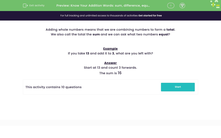# Know the Addition Words: Sum, Plus and Total

In this worksheet, students will become familiar with the vocabulary related to addition.Key stage:  KS 1

Curriculum topic:   Number: Addition and Subtraction

Curriculum subtopic:   Solve Add/Subtract Problems to 100

Difficulty level:#### Worksheet Overview

Adding whole numbers means that we are combining numbers to form a total.

We call the total in addition the sum. The sum always means addition - it is the total of when numbers are added together.

Let's look at an example:

3 + 4 = 7

The sum of 3 and 4 is 7.

Example

What is the sum of 13 and 3?

Start at 13 and count 3 forwards.

The sum of 13 and 3 is 16

Let's have a go at some questions now.### What is EdPlace?

We're your National Curriculum aligned online education content provider helping each child succeed in English, maths and science from year 1 to GCSE. With an EdPlace account you’ll be able to track and measure progress, helping each child achieve their best. We build confidence and attainment by personalising each child’s learning at a level that suits them.

Get started